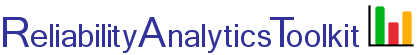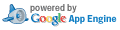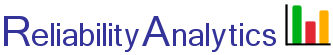# System State Enumeration, Reliability and Effective MTBF

Given a set of units in parallel and a specified minimum number of required units (m), this tool enumerates all possible states (up to a maximum of 2,000 for browser output and 10,000 for Microsoft Excel csv file output). The tool can generate the system reliability function, R(t), assuming either an exponential failure distribution (i.e., constant unit failure rate) or use the Weibull distribution to model non-constant failure rates. The tool also calculates the effective system mean time between failure (MTBF) if option "b" or "c" is selected for input #2. The effective MTBF calculation assumes that the system is restored to "as good as new" at the start of each new mission of time "T." The tool also calculates the system mean time to failure (MTTF) for the case of a non-repairable system. The specific calculation methods are described in paragraphs 2 and 3 here.

The tool can also be useful for modeling situations where there is partial "m of n" redundancy. For example, if three units are almost fully redundant (1 of 3 required), except for one critical state where units #2 and #3 both fail and unit #1 cannot deliver the full specified performance by itself, this tool allows calculating system reliability and/or effective MTBF by initially assuming full "1 of 3" redundancy, but then allowing the user to explicitly define this single state as an unsuccessful state.

Example Excel input template

## Calculation Inputs:

1. Unit list and unit reliability metric, one record per line.

The above input format is defined by selecting the appropriate pull-down in input #2 below. Example input formats for two units are:

a. Unique unit name and a unit reliability value, separated by a single space:
u1 0.97
u2 0.92

b. Unique unit name and unit failure rate, in failures per million hours, separated by a single space:
u1 52.8
u2 75.1

c. Unique unit name, Weibull shape parameter (β), Weibull characteristic life η, separated by a single spaces:
u1 1.3 40000
u2 1.8 90000

d. Unique unit name
u1
u2

As an alternative to single space delimited inputs described above, columns of data from Microsoft Excel can also be pasted into box 1.

2. Input / Output

3. Mission time (T, hours): Applicable only if failure rates or Weibull parameters are entered in box 1 above.

4. Minimum number of units required (m): Enter 0 to enumerate all possible states.

5. Output:

Send results to:
Decimal places:
Show calculation details.

6. Optional: Comma separated list of states to define as unsuccessful (e.g., 3, 5, 8):

## Featured Reference:Reliability Theory and Practice

Toolkit Home